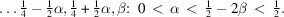International
Tables for
Crystallography
Volume B
Reciprocal space
Edited by U. Shmueli

International Tables for Crystallography (2010). Vol. B, ch. 1.5, pp. 184-188   | 1 | 2 |

## Section 1.5.5.2. Guide to the k-vector tables

M. I. Aroyoa* and H. Wondratschekb

aDepartamento de Fisíca de la Materia Condensada, Facultad de Cienca y Technología, Universidad del País Vasco, Apartado 644, 48080 Bilbao, Spain , and bInstitut für Kristallographie, Universität, D-76128 Karlsruhe, Germany
Correspondence e-mail:  wmpararm@lg.ehu.es

#### 1.5.5.2. Guide to the k-vector tables

| top | pdf |

Each figure is followed by a table with the same number. As for the figures, each table caption gives the name of the arithmetic crystal class of space groups. If there is more than one table for this arithmetic crystal class, then the symbol for the arithmetic crystal class is followed by the specific conditions for the lattice parameters, as for the figures.

Column 1. Label of the k vectors in CDML, Tables 3.9 and 3.11 and parameter description of CDML for the set of k vectors which belong to the label. No ranges for the parameters are listed in CDML.

If two k vectors belong to the same type of k vectors, then their little co-groups are conjugate under the reciprocal-space group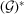and they correspond to the same Wyckoff position. Different k vectors with the same CDML label always belong to the same k-vector type. k vectors with different CDML labels may either belong to the same or to different types of k vectors. If such k vectors belong to the same type, the corresponding Wyckoff-position descriptions are preceded by the letters ex'. Frequently, such k vectors have been transformed (sign' in these tables) to equivalent ones in order to make the k vectors uni-arm, see the tables in this section.

The parameter range of a region may be described by the vertices of that region in brackets […]. One point in brackets, e.g. [P], means the point P. Two points within the brackets, e.g. [A B] means the line from A to B. Three points within the brackets, e.g. [A B C] means the triangular region of a plane with the vertices A, B and C. Four or more points may mean a region of a plane or a three-dimensional body, depending on the positions of the points. The meaning can be recognized by studying the corresponding figure. Commas between the points, e.g. [A, B, C] indicate the set {A, B, C} of the three points A, B and C.

A symbol […] does not indicate whether the vertices, boundary lines or boundary planes of the region are themselves included or not. All or part of them may belong to the region, all or part of them may not. In the parameter description of the region in Column 3 the inclusion or exclusion is stated by the symbolsor.

The backslash \' is used to indicate included parts not belonging to the described region, see e.g. the regions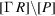and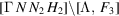in Table 1.5.5.1.

Column 2. This column describes the Wyckoff positions (given as the multiplicity, the Wyckoff letter and the site symmetry) of that symmorphic space group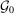of IT A which is isomorphic to the reciprocal-space group. Each Wyckoff position ofcorresponds to a Wintgen position of, i.e. to a type of k vectors ofand vice versa.

Multiplicity' is the number of points in the conventional unit cell of IT A. Here it is the number of arms of the star of the k vector, multiplied by the number of centring vectors of the conventional unit cell in IT A.

Unlike in IT A, each table starts with the Wyckoff letter a for a Wyckoff position of highest site symmetry and proceeds in alphabetical order until the general position GP is reached. The sequence of the CDML labels is not that of CDML but is determined essentially by the alphabetical sequence of the Wyckoff positions.

The symbol for the site symmetry is oriented', as given in the space-group tables of IT A. For the nomenclature, see Section 2.2.12of IT A.

Column 3. These are the parameters of that Wyckoff position ofwhich corresponds to the k-vector label in CDML, see Column 1. The parameter description and the parameter range are listed. This range is chosen such that each orbit of the Wyckoff position of IT A, i.e. also each k-vector orbit, is listed exactly once.

The following designation is used for the parameter ranges:

 (1) The statement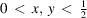means that x and y may vary independently from 0 to, 0 andboth excluded. (2) The statement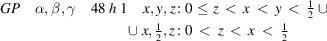means that the description of the asymmetric unit is split into two adjacent regions, a body and a plane. The boundary planeof the body is included, all other boundaries are excluded. Together the regions contain exactly one representative for each k-vector orbit of the general position GP of the reciprocal-space group. (3) The statement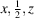: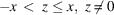means that z may assume any value between −x and +x, z = x included but z = −x and z = 0 excluded. (4) Occasionally the parameter description becomes too clumsy. Then the data listed are abbreviated by replacing the parametrical data by the designation of the corresponding region.

Example. In Table 1.5.5.3one finds for the arithmetic crystal classof space groups: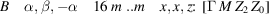The parameter description would be: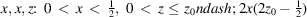Horizontal lines. The horizontal lines extending across the tables separate blocks with different numbers of free parameters. Decisive for this subdivision is the number of free parameters of the Wyckoff position to which the Wintgen position is assigned, not the number of free parameters of CDML.

#### Example. Arithmetic crystal class mm2F, see Table 1.5.5.5The k-vector labels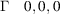' and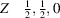' of CDML have no free parameter. However, they correspond to the Wyckoff position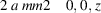', which has one free parameter. Therefore,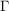andare listed together with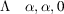' and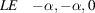' in the block for the symmetry lines, i.e. for the k vectors with one free parameter: inthere is no parameter-free Wintgen position at all.

The k-vector labels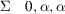' and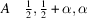' of CDML have one free parameter each. However, they correspond together with other k-vector labels to the Wyckoff position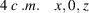'. Therefore,and A are listed together with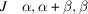' and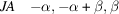' and others in the block for the planes, i.e. for the k vectors with two free parameters.

In general the sequence of the Wyckoff letters in IT A follows the falling number of free parameters. In the few cases where the sequence in IT A is different, the Wyckoff letters are exchanged. The exchange is noted at the top of the table.

Example. In the arithmetic crystal class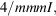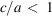, see Table 1.5.5.3, Wyckoff position e has one free parameter, whereas Wyckoff position f has constant parameters, i.e. no free parameter. Therefore, f is listed above the horizontal line, e is listed below, see Table 1.5.5.3. The note at the top of the table states Wyckoff positions e and f exchanged'.

Parameter relations. The relations between the parameters of CDML and the parameters referred to the asymmetric unit are listed at the top of the table, e.g. for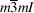in Table 1.5.5.1: `Parameter relations: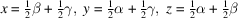'. These relations may be modified to more convenient parameters without notice, as for the plane B ofin Table 1.5.5.1: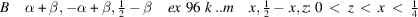instead of